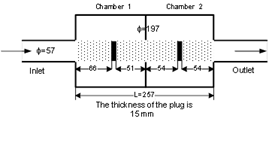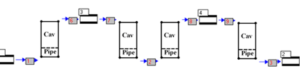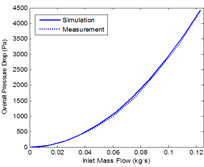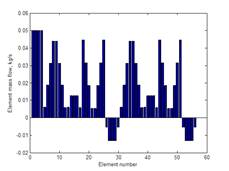#### Optical Measurement Systems (“OMS”)

This module is an add-on SIDLAB Acoustics. This is a very quick 1D tool to calculate the pressure drop introduced by the designed muffler system. It calculates the flow distribution and pressure drop inside the network using a two-port formulation, and feeds the results into the acoustic calculation in SIDLAB Acoustics. The acoustic performance of some elements like perforates is very dependent on the amount of flow inside the element. SIDLAB Flow uses the same network built in SIDLAB Acoustics.

SIDLAB Flow calculates:

Flow distribution: how much flow in kg/s is going through each element.
Pressure drop across each element in the network for the given inlet mass flow.
Flow Mach numbers in each element.
Flow – pressure drop curve for the system.

The flow can be introduced into the network in two ways:
A prescribed flow source at one inlet of the network.
Inlet flow can be defined as:
Mass flow in kg/s
Flow velocity in m/s
Flow Mach number

Several external static pressure sources connected to different inlets/outlets in the network.